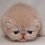# A interesting thing about exponent... does this apply to everything?

Okay... when I was solving math questions today on Brilliant, one thing just jumped out of my mind.

It is easy to see 3^{3}-1 is divisible by 2. It is not hard to see 4^{4}-1 is divisible by 3. Is 5^{5}-1 also divisible by 4? Is 6^{6}-1 also divisible by 5?

Moreover, for example, is 17^{15}-1, 17^{16}-1, 17^{17}-1, 17^{18}-1 all divisible by 16?

I think it is. Here is my proof:

Let a and n be positive integers.

a^{n} =(a-1)(a^{n-1})+a^{n-1} =(a-1)(a^{n-1}+(a-1)(a^{n-2})-(a^{n-2}) =(a-1)(a^{n-1}+(a-1)(a^{n-2})-(a^{n-2})+......(a-1)a+(a-1)+1 after factoring, we get (a-1)(a^{n-1}+a^{n-2}+a^{n-3}+......+1)+1 therefore, a^{n}-1 =(a-1)(a^{n-1}+a^{n-2}+a^{n-3}+......+1)+1-1 =(a-1)(something)

and it is divisible by a-1.

But now I have another question. Does similar theory also apply to negative integers? (for example, let n be a negative integer)

Help me please! Thank you very much!

(P.S. I only learned Algebra 1 so sometimes I could be confused to use or even look at some notations. Thank you for your tolerance!)Note by Margaret Zheng
6 years, 1 month ago

This discussion board is a place to discuss our Daily Challenges and the math and science related to those challenges. Explanations are more than just a solution — they should explain the steps and thinking strategies that you used to obtain the solution. Comments should further the discussion of math and science.

When posting on Brilliant:

• Use the emojis to react to an explanation, whether you're congratulating a job well done , or just really confused .
• Ask specific questions about the challenge or the steps in somebody's explanation. Well-posed questions can add a lot to the discussion, but posting "I don't understand!" doesn't help anyone.
• Try to contribute something new to the discussion, whether it is an extension, generalization or other idea related to the challenge.
• Stay on topic — we're all here to learn more about math and science, not to hear about your favorite get-rich-quick scheme or current world events.

MarkdownAppears as
*italics* or _italics_ italics
**bold** or __bold__ bold
- bulleted- list
• bulleted
• list
1. numbered2. list
1. numbered
2. list
Note: you must add a full line of space before and after lists for them to show up correctly
paragraph 1paragraph 2

paragraph 1

paragraph 2

[example link](https://brilliant.org)example link
> This is a quote
This is a quote
    # I indented these lines
# 4 spaces, and now they show
# up as a code block.

print "hello world"
# I indented these lines
# 4 spaces, and now they show
# up as a code block.

print "hello world"
MathAppears as
Remember to wrap math in $$ ... $$ or $ ... $ to ensure proper formatting.
2 \times 3 $2 \times 3$
2^{34} $2^{34}$
a_{i-1} $a_{i-1}$
\frac{2}{3} $\frac{2}{3}$
\sqrt{2} $\sqrt{2}$
\sum_{i=1}^3 $\sum_{i=1}^3$
\sin \theta $\sin \theta$
\boxed{123} $\boxed{123}$

## Comments

Sort by:

Top Newest

To check, do you know what Modular Arithemtic is? How about the Binomial Theorem?

Staff - 6 years, 1 month ago

Log in to reply

I know binomial theorem, but I'm not sure with modular arithmetic. And thank you very much!

- 6 years, 1 month ago

Log in to reply

×

Problem Loading...

Note Loading...

Set Loading...